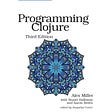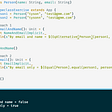Scala:Railway oriented programming primer

Railway oriented programming is a two-track style of chaining functions together, with the green representing the success track and red representing the failure track. This post is to explore why this style came into being.

One of the ideals in the functional programming world is the concept of total functions where total functions are functions that would return the correct output value for every possible input that it can take. This specifically means that the function should not throw exceptions. If a function can throw exceptions, then it is no longer a total function. From the consumer’s perspective, it can no longer be sure that the function would always return the right result. So, we aspire to create total functions that just always works.

However, that is not always possible as there can be error conditions. That is where an abstraction that represents a tuple with either the output or the error comes in. Either is the abstraction.

Now, you feel equipped to represent real worldly situations much better and your functions also achieve the ideal of a total function. Once you have functions returning eithers, the clients of these functions would start handling the success result and the failure results separately. In a system, you will tend to have a chain of hundreds of functions which handle left and right of the eithers. That does indeed look like a railway network.

A simple function returning an Either as below. String on the left is used to capture the error condition.

`def doubleIfEven(n : Int) : Either[String,Int] ={  if(n % 2 == 0)       Right( n * 2)  else       Left("Odd number")}`

If the caller calls this function with a “good” argument, he gets a Right.

`println(doubleIfEven(2))Right(4)`

If the caller calls this function with a “bad” argument, he gets a Left.

`println(doubleIfEven(1))Left(Odd number)`

To extract the actual value from within the either, use a map function. Assignments are not ideal in the functional programming world.

`doubleIfEven(2).map(println)4`

If the condition was a failure, the map would just not execute.

`doubleIfEven(1).map(println)No output`

A simple right to right chaining would look as below

`def doubleIfEven(n : Int) : Either[String,Int] ={  if(n % 2 == 0)       Right( n * 2)  else       Left("Odd number")}def doubleIfOdd(n : Int) : Either[String, Int] = {  if( n % 2 == 1)    Right(n * 2)  else     Left("Even number")}doubleIfOdd(1)  .map(r => doubleIfEven(r).map(println))4`

Here, the green track from the `doubleIfOdd` is continuing through the green track of the `doubleIfEven` . So, the input of 1 comes out of the green track of `doubleIfOdd` as 2 and serves as the input of `doubleIfEven` and comes out of the green track of `doubleIfEven` as 4.

Error flows

If the first input is even, then the flow does not continue at all as shown below.

`doubleIfOdd(2)  .map(doubleIfEven)   .map(println)*No ouput*`

Passes through first function but not through the second function

`doubleIfOdd(1)   .map(doubleIfSixFold)   .map(println)Left(Not a six fold number)`

--

--

--

## More from Learn

On a continuing learning journey..

## Objective: Use Fractional Numbers Instead of Integers## Clojure Coding Quick Start## You Don’t Have to Feel Trapped## Continuous Integration for .Net with Jenkins and NuGet## I accidentally became a self-taught web developer## Modeling GraphQL Mutations## Learn

On a continuing learning journey..

## Scala: Partial function argument in collect api## Learn Functional Programming With Me — Part 1## Type Classes in Scala 2## Clojure Programming: A deep dive into fundamentals